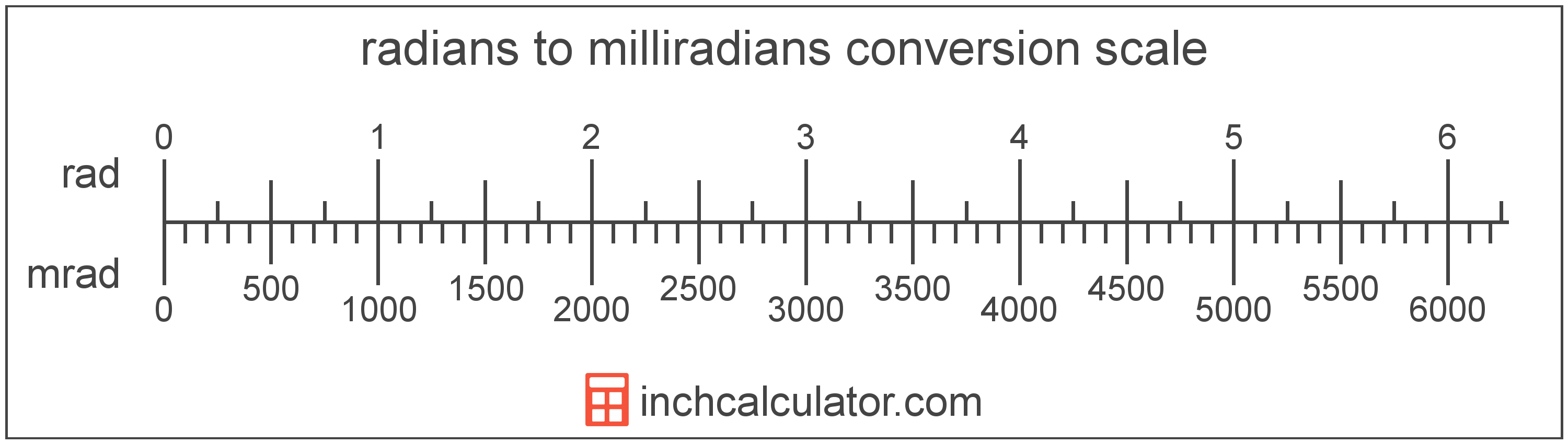Enter the angle in milliradians below to get the value converted to radians.To convert a milliradian measurement to a radian measurement, divide the angle by the conversion ratio.

Since one radian is equal to 1,000 milliradians, you can use this simple formula to convert:

The angle in radians is equal to the milliradians divided by 1,000.

For example, here's how to convert 5,000 milliradians to radians using the formula above.

A milliradian is equal to 1/1,000 of a radian. One degree is equal to 0.0573 milliradians and there are 6,283 milliradians in a full revolution/circle. The milliradian is also a commonly used unit in mathematics.

The milliradian is a multiple of the radian, which is the SI derived unit for angle. In the metric system, "milli" is the prefix for 10-3. A milliradian is sometimes also referred to as a mil. Milliradians can be abbreviated as mrad; for example, 1 milliradian can be written as 1 mrad.

A radian is the measurement of angle equal to the start to the end of an arc divided by the radius of the circle or arc. 1 radian is equal to 180/π, or about 57.29578°. There are about 6.28318 radians in a circle.

The radian is the SI derived unit for angle in the metric system. Radians can be abbreviated as rad, and are also sometimes abbreviated as c, r, or R. For example, 1 radian can be written as 1 rad, 1 c, 1 r, or 1 R.

Radians are often expressed using their definition. The formula to find radians is θ = s/r, where the angle in radians θ is equal to the arc length s divided by the radius r. Thus, radians may also be expressed as the formula of arc length over the radius.